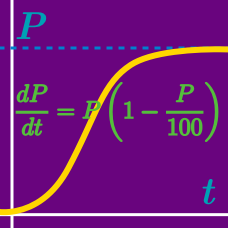Calculus

# Differential Equations - dy/dx = f(x)

If function $f(x)$ satisfies $\frac{df(x)}{dx}=(3x+4)(2-x) \text{ and } f(-1)=18,$ what is $f(x)?$

If $f'(x)=9x^2$ and $f(2)=31,$ what is $f(x)?$

If $f'(x)=\sin x-2\cos x, f(\pi)=9,$ what is $f(x)?$

Suppose that the acceleration $a(t)$ of a particle at time $t,$ is given by the formula $a(t)=4t-3.$ If $v(1)=8, f(2)=5,$ where $v(t)$ and $f(t)$ are the velocity and position function of the particle, respectively, what is the position of the particle when $t=1 ?$

The velocity at time $t$ of a particle moving along the $x$-axis is given by the formula $v(t)=8t^3-9t^2.$ If the initial position of the particle on the $x$-axis is $x=9$, what is its position when $t=2 ?$

×